Ellipse Based Shape Parameters edit page

In this section we discuss geometric properties of grains that are related to ellipses fitted to the grains. Additionally to the orientation omega, and the lengths a, b of the long axis and short axes that are computed by the command [omega,a,b] = grains.fitEllipse the following properties based on the fitted ellipses are avaiable.

 long axis as vector3d short axis as vector3d midpoint long axis / short axis

In order to demonstrate these properties we start by reconstructing the grain structure from a sample EBSD data set.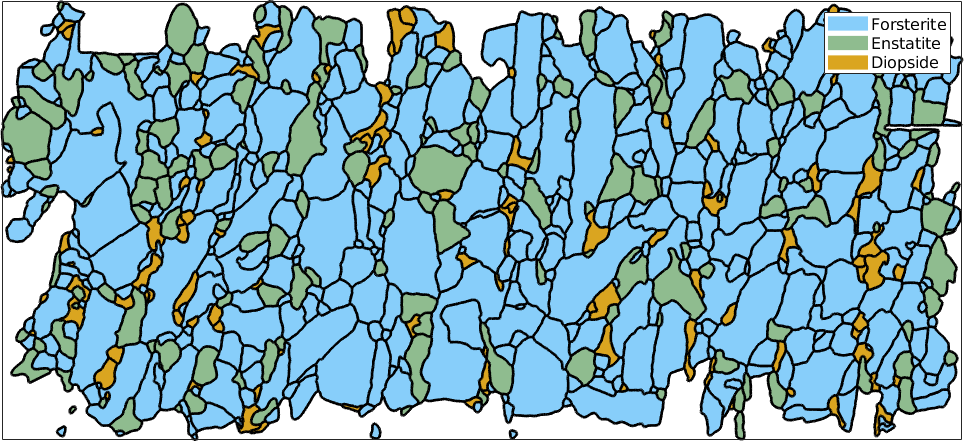## Fit Ellipses

The basic command for fitting ellipses is fitEllipse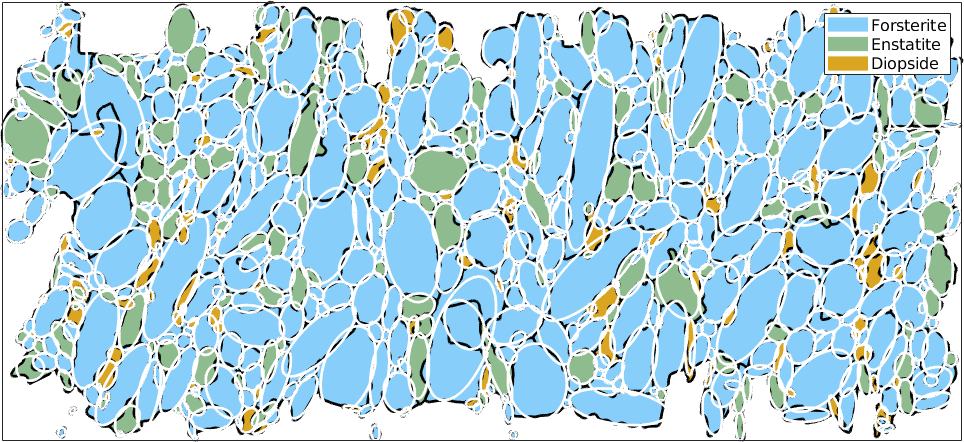The returned variable omega is the angle describing the rotation of the ellipses and a and b are the length of the longest and shortest half axis. The midpoints of the ellipses can be computed by the command grains.centroid. Note, that the ellipses are scaled such that the area of the ellipse coincides with the actual grain area. Alternatively, one can also scale the ellipse to fit the boundary length by using the option boundary.

## Long and Short Axes

The direction of the long and the short axis of the fitted ellipse can be obtained by the comands grains.longAxis and grains.shortAxis. These directions are only well defined if the fitted ellipse is not to close to a perfect circle. A measure for how distinct the ellipse is from a perfect circle is the aspect ratio which is defined as the quotient $$a/b$$ between the longest and the shortest axis. For a perfect circle the apect ratio is $$1$$ and increases to infinity when the ellipse becomes more and more elongated.

Lets colorize the grains by their apect ratio and plot on top the long axis directions: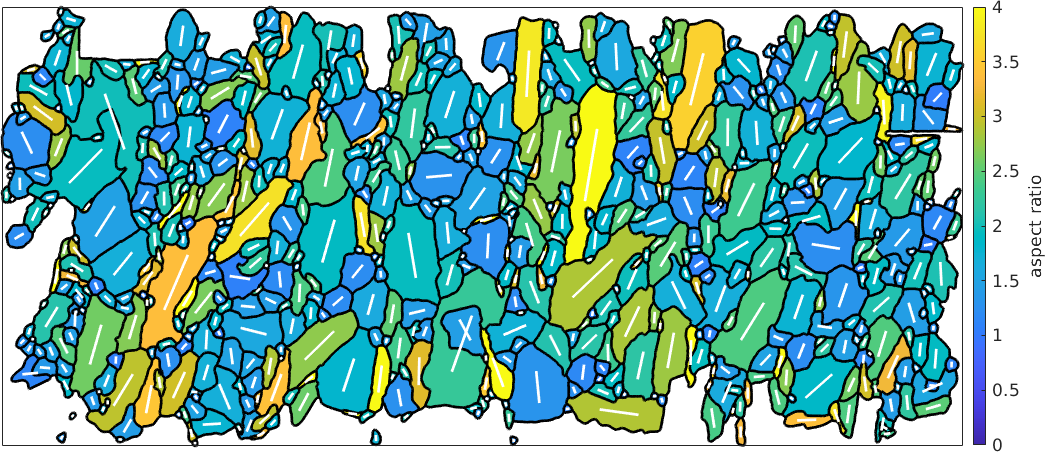## Shape perfered orientation

If we look at grains, we might wonder if there is a characteristic difference in the grain shape fabric between e.g. Forsterite and Enstatite. In contrast to crystal prefered orientations which which describe on the alignment of the atome lattices the shape prefered orientation (SPO) describes the algnment of the grains by shape in the bulk fabric.

Long Axis Distribution

The most direct way to analyse shape prefered orientations are rose diagrams of the distribution of the grain long axes. For those diagrams it is useful to weight the long axis by the grain area such that larger grains have a bigger impact on the distribution and by the aspect ratio as for grains with a small aspect ratio the long axis is not so well defined.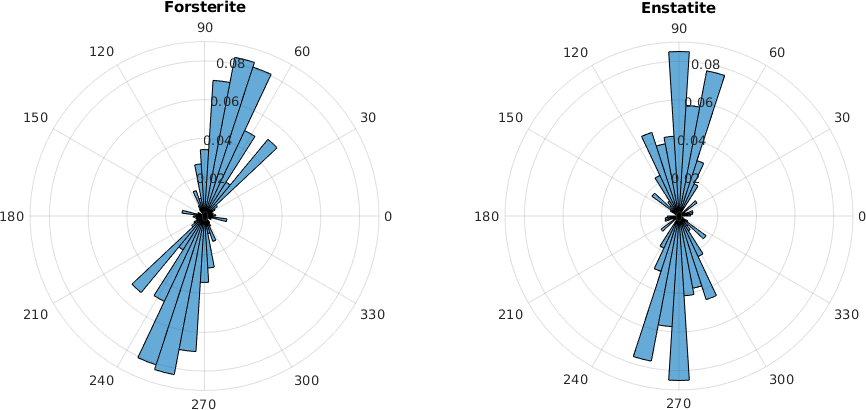Instead of the histogram we may also fit a circular density distribution to the to the long axes using the command calcDensity.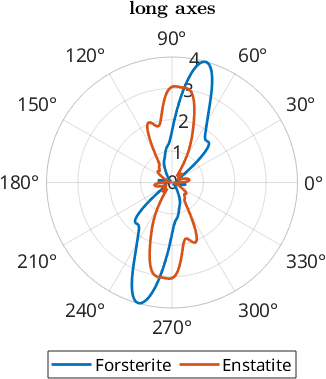## Shortest Caliper Distribution

Alternatively, we may wonder if the common long axis of grains is does suitably represented by the direction normal to the shortest caliper of the grains. This can particularly be the case for aligned rectangular particles. The command calcTDF also takes a list of angles and a list of weights or lengths as input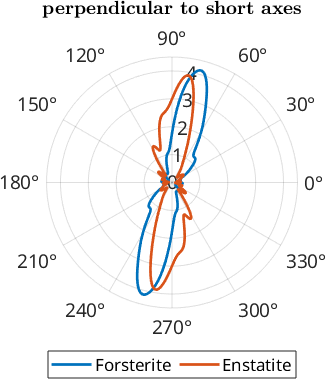We can also smooth the functions with a wrapped Gaussian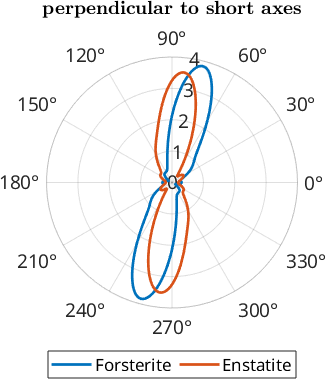Because best fit ellipses are always symmetric and the projection function of an entire grain always only consider the convex hull, grain shape fabrics can also be characterized by the the length weighted rose diagram of the directions of grain boundary segments.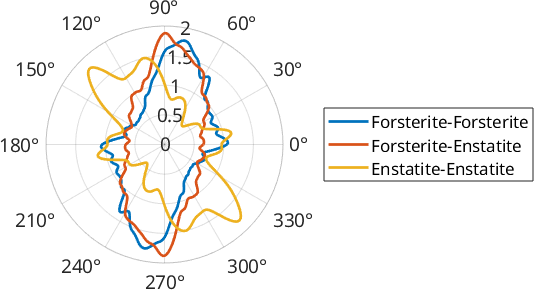## Characteristic Shape

Note that this distribution is very prone to inherit artifacts based on the fact that most EBSD maps are sampled on a regular grid. We tried to overcome this problem by heavily smoothing the grain boundary. The little peaks at 0 and 90 degree are very likely still related to this sampling artifact.

If we just add up all the individual elements of the rose diagram in order of increasing angles, we derive the characteristic shape. It can be regarded as to represent the average grain shape.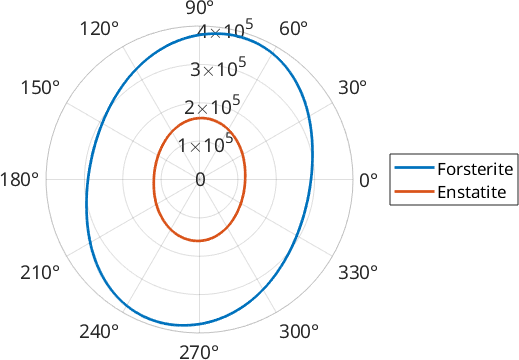We may wonder if these results are significantly different or not TODO: get deviation from an ellipse etc